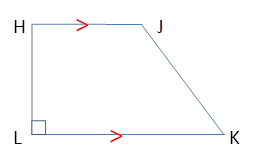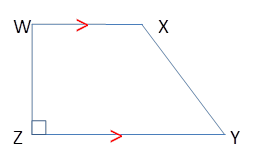Chapter 4.4, Problem 28EElementary Geometry For College St...

7th Edition
Alexander + 2 others
ISBN: 9781337614085

Solutions

Chapter
SectionElementary Geometry For College St...

7th Edition
Alexander + 2 others
ISBN: 9781337614085
Textbook Problem

HJKL and WXYZ are right trapezoids. Are these trapezoids congruent ifa) H L ¯ ≅ W Z ¯             a n d             L K ¯ ≅ Z Y ¯ ? b) H L ¯ ≅ W Z ¯     ,       L K ¯ ≅ Z Y ¯ ,               a n d           ∠ K ≅ ∠ Y ?To determine

To Find:

Whether the given trapezoids are congruent.

Explanation

The given trapezoids HJLK and WXYZ are

We know that for two right trapezoids to be congruent, the base angles of both the trapezoids needs to be congruent.

We know that for right trapezoid HJ¯||LK¯andLH.

Similarly WX¯||ZY¯a

To determine

To Find:

Whether the given trapezoids are congruent.

Still sussing out bartleby?

Check out a sample textbook solution.

See a sample solution

The Solution to Your Study Problems

Bartleby provides explanations to thousands of textbook problems written by our experts, many with advanced degrees!

Get Started

Find more solutions based on key concepts